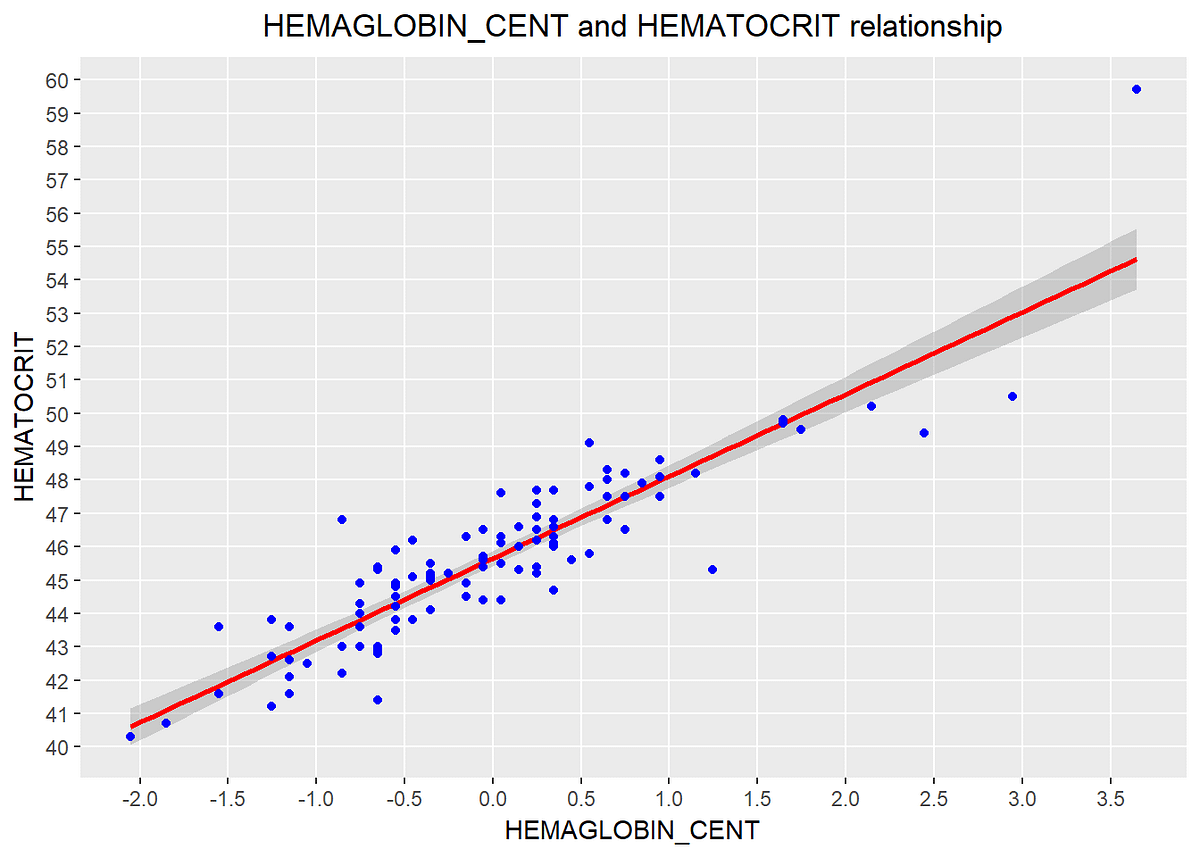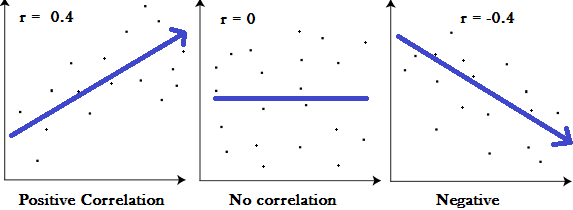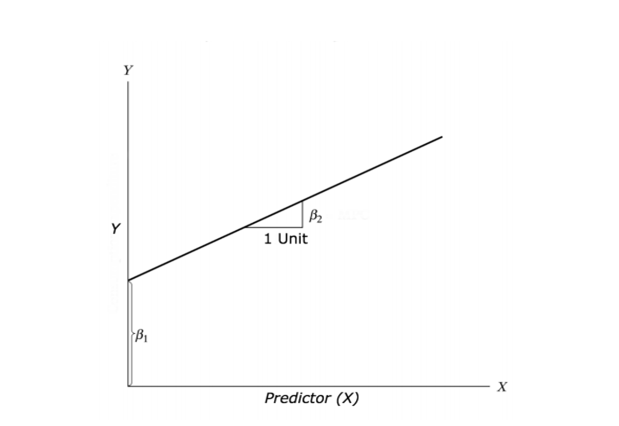# Correlation and Regression: A Case Study in RCorrelation is a statistical measure that indicates the extent to which two or more variables fluctuate together. Positive Correlation indicates the extent to which those variable increase.

## Introduction :

*Correlation *is a statistical measure that indicates the extent to which two or more variables fluctuate together. *Positive Correlation *indicates the extent to which those variable increase or decrease in parallel; *Negative Correlation *indicates the extent to which one variable increases as the other decreases.

### Correlation Coefficient :

1. A correlation coefficient is a way to put a value to the relationship.
2. Correlation Coefficient has a value between** -1 and 1**
3. A “0” means there is no relationship between the variables at all.
4. While *-1 or 1 *means that there is a perfect negative or positive correlation.

### Example :### Regression Analysis :

It is a Statistical method To determine whether there is a relationship between two variables.

Response variable and the explanatory variable are continuous variables (i.e. real numbers with decimal places — things like heights, weights, volumes, or temperatures).

### Definition:

To predict the value of an outcome variable Y based on one or more input predictor variables X.

Aim: To establish a linear relationship (a mathematical formula) between the predictor variable(s) and the response variable, so that, we can use this formula to estimate the value of the response Y, when only the predictors (Xs) values are known.

## Y = β1 + β2X + ε

• β1 is the intercept and β2 is the slope.

• Collectively, they are called regression coefficients.

• _ε _is the error term, the part of _Y _the regression model is unable to explain.## 7 steps to run a linear regression analysis using R

7 steps to run a linear regression analysis using R. I learned how to do regression analysis in R using brute force. With these 7 copy and paste steps, you can too.

## Data Manipulation In R | Data Manipulation In R With dplyr | R Programming For Beginners

This video on Data Manipulation in R will help you learn how to transform and summarize your data using different packages and functions. You will use the dplyr package to select, filter, arrange, and mutate data. You will use the tidyr library to create tidy data. You will look at functions such as gather, spread, separate, and unite. Let's begin!

## GGPlot2 In R Tutorial | GGPlot2 Basics | Data Visualization In R | R Programming

This video on GGplot Tutorial will help you understand one of R's most widely used data visualization libraries. You will learn how to create different charts such as bar graphs, stacked bar charts, histograms, scatter plots, box plots, and lots more. You will get to know the different functions that ggplot library provides, such as geom_bar, geom_point, geom_line, geom_violin, and others. Let's get started.

## Using regression with correlated data

Using regression with correlated data. Tutorial (including R code) for using Generalized Estimating Equations and Multilevel Models.

## R For Data Science Full Course | Data Science With R Full Course |Data Science Tutorial

Learn the essential concepts in data science and understand the important packages in R for data science. You will look at some of the widely used data science algorithms such as Linear regression, logistic regression, decision trees, random forest, including time-series analysis. Finally, you will get an idea about the Salary structure, Skills, Jobs, and resume of a data scientist.